The Pythagorean Theorem and its Converse

Using the Pythagorean Theorem

Example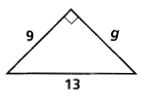Using the Pythagorean Theorem, substitute g and 9 for the legs and 13 for the hypotenuse.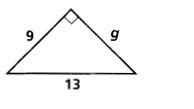a2 + b2 = c2

g2 + 92 = 132           Substitute

g2 + 81 = 169           Simplify

g2 = 88             Subtract 81 from each side

g2 =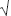88           Take the square root

g =  2(22)      Simplify

You can also use the Pythagorean Theorem in the other direction (that is, use the converse of the Pythagorean Theorem) to determine whether a triangle is right.

Example

Is a triangle with sides of lengths 8, 12, and 14 a right triangle?

If this is a right triangle, then the sides should follow the Pythagorean Theorem, with the longest side being the hypotenuse.
a2 + b2 = c2

82 + 122 ? 142

64 + 144 ? 196

208 > 196

The theorem doesn't hold.  So the triangle is not a right triangle.

As a bonus, however, we can figure out what kind of triangle this is.

If a2 + b2 > c2, the triangle is acute.

If a2 + b2 < c2, the triangle is obtuse.

Because 208 > 196, the triangle is acute.

Guided Practice

1.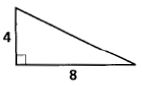2.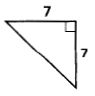3.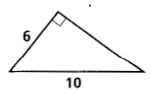4.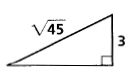5.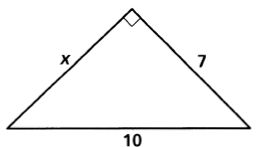6.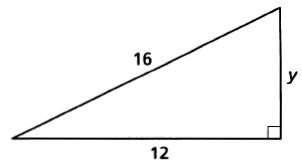7.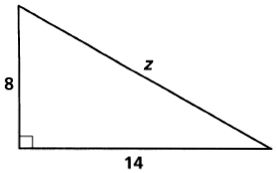8.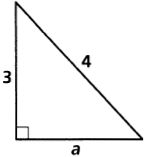9.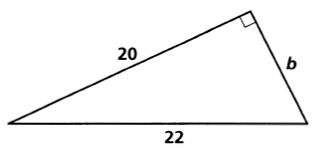10.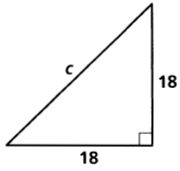11.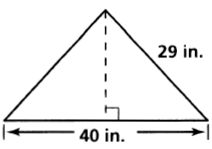12.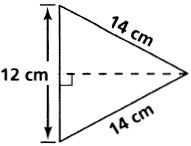13.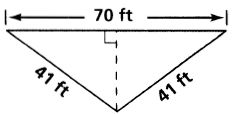14.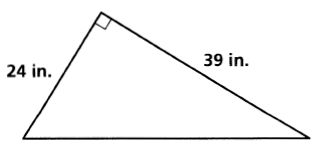15.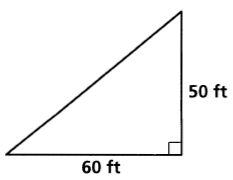16.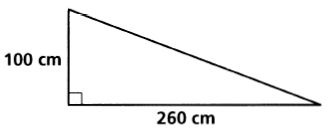17.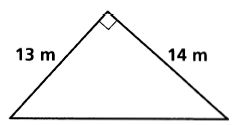The numbers represent the lengths of the sides of a triangle. Classify each triangle as acute, obtuse, or right.

18.       6, 9, 10

19.       7, 24, 25

20.       18, 24, 30

21.       2, 5, 6

22.       20, 100, 110

23.       13, 21, 24

1.  45

2.  72

3.  8

4.  6

5.51

6.  47

7.  265

8.7

9.  221

10.  182

11.  420 in2

12.  2410 cm2

13.  70114 ft2

14.  468 in2

15.  1500 ft2

16.  13,000 cm2

17.  91 m2

18.  acute

19.  right

20.  right

21.  obtuse

22.  obtuse

23.  acute